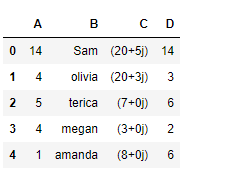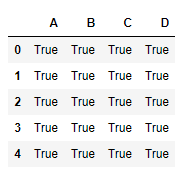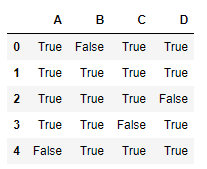# Python | Pandas dataframe.notnull()

• Last Updated : 25 Aug, 2021

Python is a great language for doing data analysis, primarily because of the fantastic ecosystem of data-centric python packages. Pandas is one of those packages and makes importing and analyzing data much easier.
Pandas dataframe.notnull() function detects existing/ non-missing values in the dataframe. The function returns a boolean object having the same size as that of the object on which it is applied, indicating whether each individual value is a na value or not. All of the non-missing values gets mapped to true and missing values get mapped to false.
Note : Characters such as empty strings ” or numpy.inf are not considered NA values. (unless you set pandas.options.mode.use_inf_as_na = True).

Syntax: DataFrame.notnull()
Returns : Mask of bool values for each element in DataFrame that indicates whether an element is not an NA value.Example #1: Use notnull() function to find all the non-missing value in the dataframe.

## Python3

 `# importing pandas as pd``import` `pandas as pd` `# Creating the first dataframe``df ``=` `pd.DataFrame({``"A"``:[``14``, ``4``, ``5``, ``4``, ``1``],``                   ``"B"``:[``"Sam"``, ``"olivia"``, ``"terica"``, ``"megan"``, ``"amanda"``],``                   ``"C"``:[``20` `+` `5j``, ``20` `+` `3j``, ``7``, ``3``, ``8``],``                   ``"D"``:[``14``, ``3``, ``6``, ``2``, ``6``]})` `# Print the dataframe``df`Let’s use the dataframe.notnull() function to find all the non-missing values in the dataframe.

## Python3

 `# find non-na values``df.notnull()`

Output :As we can see in the output, all the non-missing values in the dataframe has been mapped to true. There is no false value as there is no missing value in the dataframe

Example #2: Use notnull() function to find the non-missing values, when there are missing values in the dataframe.

## Python3

 `# importing pandas as pd``import` `pandas as pd` `# Creating the dataframe``df ``=` `pd.DataFrame({``"A"``:[``"Sandy"``, ``"alex"``, ``"brook"``, ``"kelly"``, np.nan],``                   ``"B"``:[np.nan, ``"olivia"``, ``"terica"``, "``", "``amanda"],``                   ``"C"``:[``20` `+` `5j``, ``20` `+` `3j``, ``7``, ``None``, ``8``],``                    ``"D"``:[``14.8``, ``3``, ``None``, ``2.3``, ``6``]})` `# find non-missing values``df.notnull()`

Output :Notice, the empty string also got mapped to true indicating that it is not a NaN value.

My Personal Notes arrow_drop_up